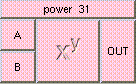POWERTHE BOX'S MAIN HELP: Result of this function is A raised to the power of B; Example: pow(2,3.0) = 2*2*2 = 8; Example: pow(4,0.5) = sqrt(4) = 2; A (input): This value must be a scalar. B (input): Input A is raised to the power of this value. This value must be a scalar. OUT (output): Result is A raised to the power of B; Example: pow(2,3.0) = 2*2*2 = 8; Example: pow(4,0.5) = sqrt(4) = 2;

<-- BACK TO Math